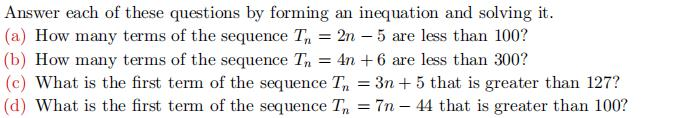### Still have math questions?

Algebra
QuestionAnswer each of these questions by forming an inequation and solving it.

(a) How many terms of the sequence $$T _ { n } = 2 n - 5$$ are less than $$100 ?$$

(b) How many terms of the sequence $$T _ { n } = 4 n + 6$$ are less than $$300 ?$$

(c) What is the first term of the sequence $$T _ { n } = 3 n + 5$$ that is greater than $$127 ?$$

(d) What is the first term of the sequence $$T _ { n } = 7 n - 44$$ that is greater than $$100$$ ?

$$\left. \begin{array} { l l l l } { \text { (a) } 52 } & { \text { (b) } 73 } & { \text { (c) } T _ { 41 } = 128 } & { \text { (d) } T _ { 21 } = 103 } \end{array} \right.$$